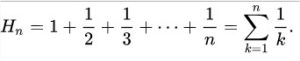# Java Code to Calculate Harmonic Value | Java Programs

Java program to calculate harmonic value – In this article, we will learn the multiple ways to calculate the harmonic value in Java Programming.

Suitable examples and sample programs have been included in order to make you understand simply. The compiler has also been added so that you can execute the programs yourself.

• Using Standard Method
• Using Scanner Class
• Using Static Method
• Using Separate Class

Harmonic Value or the Harmonic Number is the sum of the reciprocals up to a given value.TWe can see that this is the general formula to calculate the Harmonic Value for the first n numbers.

If n = 4, then

Hn = 1 + 1/2 + 1/3 + 1/4 = 2.083333

## Harmonic Value – Using Standard Method

To determine the harmonic value, our first step is to get input of the number until which we want to find the harmonic value. The input value can be assigned to a variable (n) in the code itself and our task in to find the harmonic value from 1 to this number.

In order to store the harmonic value, we take another variable (sum). Then we iterate using a loop till the number and keep adding all the reciprocal numbers as shown below:

for( i=1;i<=n;i++) { sum=sum+(1/i); }

This value sum, is our resultant output harmonic value, which can be displayed in the output screen as desired.

Output:

### Using Scanner Class

In the standard method, the input for the program was given in the code itself. In future, if we want to try the same code for a different input then, it’ll require us to go the code and make the necessary changes in the input. This is not a convenient method.

Instead, we could make use of a special class in Java named the Scanner class. By making use of this class, inputs for any primitive datatype like int, float, double, char, etc., can be read at runtime itself in the console screen by the user.

Thereby, making use of this scanner class, we’ll read our required input a number (n) of type double and the calculate the sum of reciprocals from 1 to the number (n).

Output1:

Using Static Method

In both the previous methods, we have seen that the entire code is written inside main method only.

The problem that arises with this is that, when we would like to make use of the same logic again in the code, we’ll have to rewrite the same set of statements.

Instead of doing so, if we write the logic used within another static method, it saves a lot of time, reduces number of lines as we’ll just have to call this method and pass necessary parameters wherever required. This way a part of code becomes reusable.

Output1:

##### Using Separate Class

In all the above method, the entire code is written inside the same class.

The problem that arises with this is that, the code when written like that is not that readable. It could be difficult to find a particular part of code. Instead, we can split the code and make use of another separate class.

To do so, we’ll split the code into two parts. The first part is the main method class which is responsible for all the input output operations same as the previous methods while, the second part is another separate class(Hvalue) which will contain a constructor or method having the same logic as discussed above.

This will be invoked with the help of an object created referencing it (h) within the main method.

x

## Java Program to Add Two Matrices – 4 Ways | Programs

Java program to add two matrices – The following Java Code will let you know ...